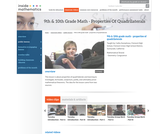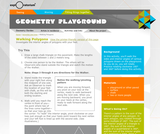Updating search results...

# 2 Results

View
Selected filters:قراءة النص المطبوع بخط رفيع
Rating
0,0 stars

This lesson is about properties of quadrilaterals and learning to investigate, formulate, conjecture, justify, and ultimately prove mathematical theorems. Students will: Analyze characteristics and properties of two- and three-dimensional geometric shapes; develop mathematical arguments about geometric relationships; and apply appropriate techniques, tools, and formulas to determine measurements.Explore relationships among classes of two- and three-dimensional geometric objects, make and test conjectures about them, and solve problems involving them. Employ forms of mathematical reasoning and proof appropriate to the solution of the problem at hand, including deductive and inductive reasoning, making and testing conjectures, and using counter examples and indirect proof. Identify, formulate and confirm conjectures. Establish the validity of geometric conjectures using deduction, prove theorems, and critique arguments made by others. (9th/10th Grade Math)

نوع المادة:
Activity/Lab
Lecture
Lesson Plan
Teaching/Learning Strategy
Provider:
Noyce Foundation
Provider Set:
في قلب الرياضيات
المؤلف:
Humphreys, Cathy
11/30/2011Conditional Remix & Share Permitted
CC BY-NC-SA
Rating
0,0 stars

In this activity, learners walk the sides and interior angles of various polygons drawn on the playground. As they do so, learners practice rotating clockwise 180 and 360 degrees. Learners discover there is a pattern to the sum of the interior angles of any polygon.

نوع المادة:
Activity/Lab
Provider:
متحف Exploratorium لدعم التعليم
المؤلف:
Exploratorium
Gordon and Betty Moore Foundation
National Science Foundation
The Exploratorium